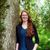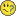# QlikView App Dev

Discussion Board for collaboration related to QlikView App Development.

Announcements
QlikWorld 2022, LIVE in Denver CO., May 16-19, 2022. REGISTER NOW TO RECEIVE EARLY BIRD PRICING
cancel
Showing results for
Did you mean:Not applicable

## Accumulation in Pivot Table

Hi everyone,

I’m trying to accumulate some values inside a Pivot Table (so I can use horizontal dimensions). The challenge is that I need to accumulate month by month only certain values whilst using non-accumulated values for the second part of the expression.

I’m attaching a sample file in which the example will become clearer.

20 RepliesCreator III

How to sum across rows in a pivot table (running total)

Attached is how to do it with your example .Not applicable
Author

Hello Michael, ive already try using the function "Before" but it wont work because i need to sum the accumulation of values "Type A" and then sum the corresponding "Value B"MVP

From what I'm seeing, it looks like you can't do it in one expression.  In my attached file, when I calculate the accumulation of A and just the sum of B in two different expressions, then add those two together, it works fine.  The problem comes in when I try to put the two expressions into one.  Maybe someone else can chime in if they have any ideas because I'm stumped.Not applicable
Author

Thanks Nicole but that is exactly the problem i need them to be in just one expressionCreator III

Try the attached.  I mapped the months to periods.  That way you can multiply the month by Value A and add Value B.

So Jan = 1 so   1*Value A +Value B

Feb = 2 so  2*Value A +Value B

and so on.Partner

Try a formula like this;

This works Left to right. Pivot table

RangeSum(Before(Sum(YourField),0, ColumnNo()))

Top to bottom

RangeSum(Above(Sum(YourField),0, RowNo()))MVP

Got it!

 =RangeSum(Before(sum({\$} Value),1,12), sum({\$} Value))+sum({\$} Value)

Also see the attached.

EDIT:  I forgot to mention that the 1,12 in the before() is accumulating 12 steps back (1 year), as I wasn't sure on your exact requirement.  If you want to accumulate over everything, replace the 12 with ColumnNo(TOTAL).Employee

I´m not sure if I understand you requirement, but try this:

```rangesum(
sum({\$<Type={'Value B'}>} Value),
Before(
sum({\$<Type={'Value A'}>} Value),
0,
ColumnNo(TOTAL)
)
)
```Creator III

Well your solution is more elegant than mine as usualCommunity Browser